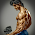### Entropy

Entropy is an important thermodynamic property of a working substance, which increases with the addition of heat; and decreases with its removal. As a matter of fact, it is tedious to the term Entropy. But it is comparatively easy to define change of entropy of a working substance. Over small range of temperature, the increase or decrease of entropy, when multiplied by the absolute temperature, gives the heat absorbed or rejected by the working substance. Mathematically, heat absorbed or rejected by the working substance,

δQ = T dS

where

T = Absolute temperature, and
dS = Increase or decrease in entropy.

Theoretically, the entropy of a substance is zero at absolute zero temperature. Hence, in entropy calculations, some convenient datum should be selected from which measurement may be made.

It may be noted that water at 0° C is assumed to have zero entropy, and changes in its entropy are reckoned from this temperature.

1.What is the entropy of universe then..?explain

1.entropy of universe is increasing day by day

2.Same of my note book cope ,so thankful to you

3.Same of my note book copy, so thankful to you